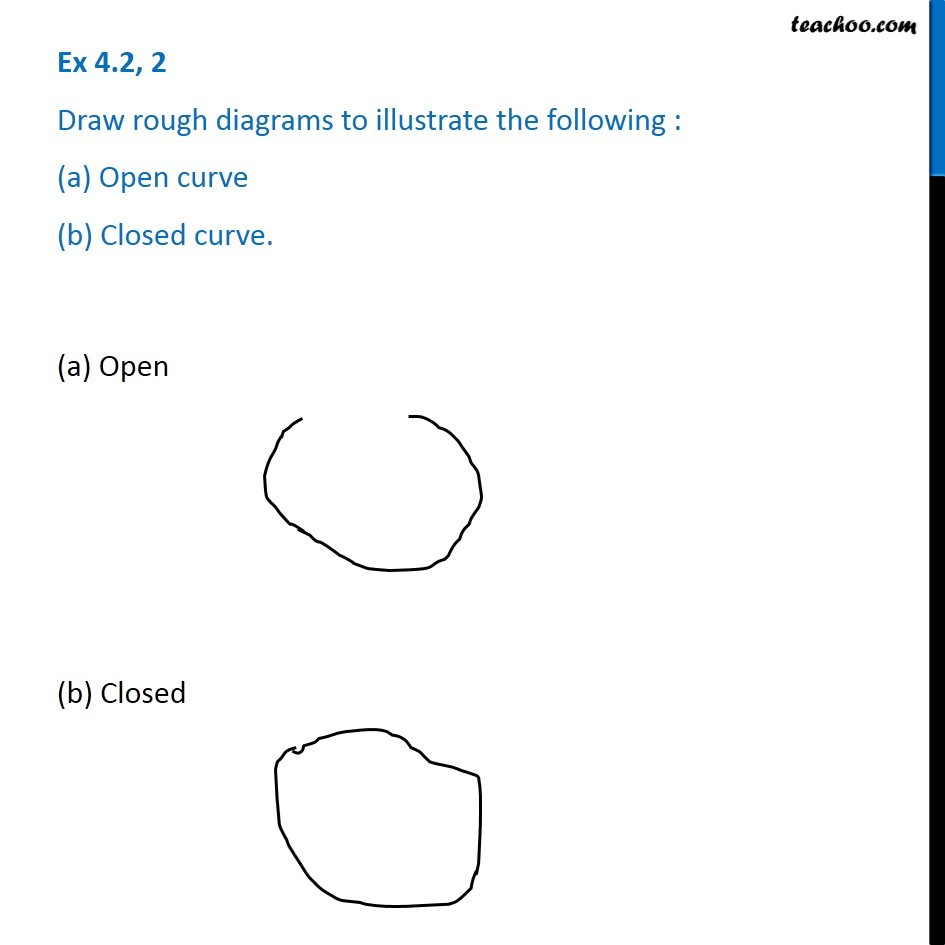Curve, Polygons

Chapter 4 Class 6 Basic Geometrical Ideas
Concept wiseLearn in your speed, with individual attention - Teachoo Maths 1-on-1 Class

### Transcript

Ex 4.2, 2 - Chapter 4 Class 6 NCERT Draw rough diagrams to illustrate the following: (a) Open curve (b) Closed curve. A open curve is a figure with its ends open, A closed figure is a curve with its end closed. The examples of both are given in the diagram